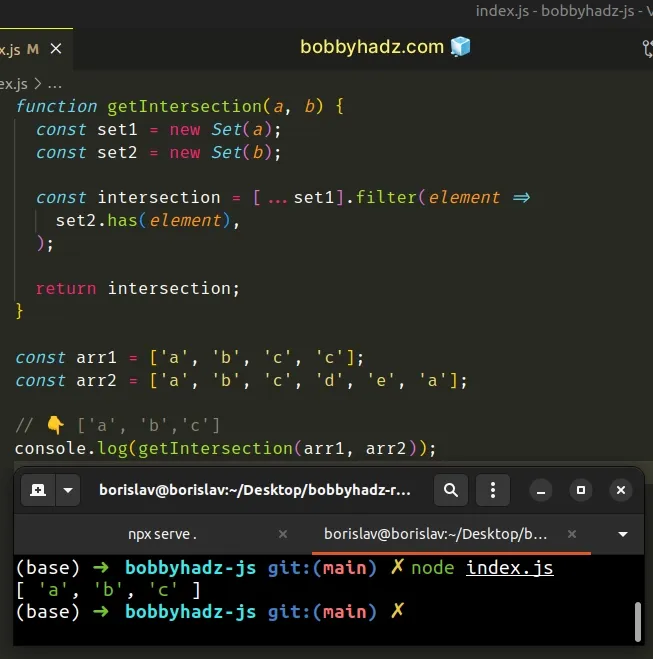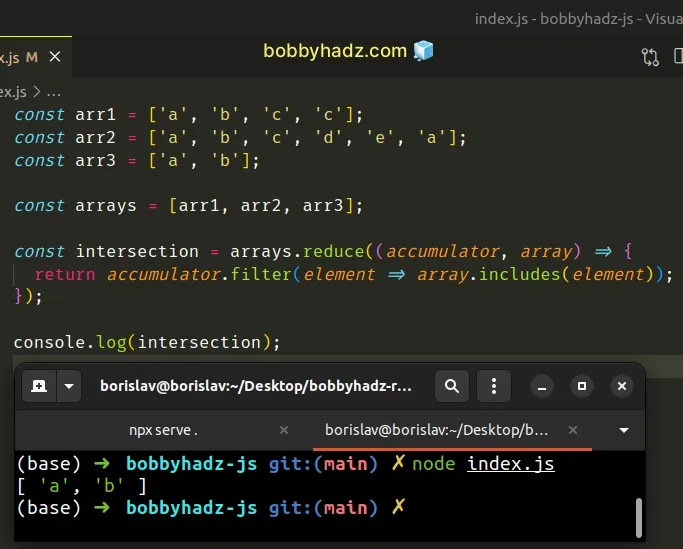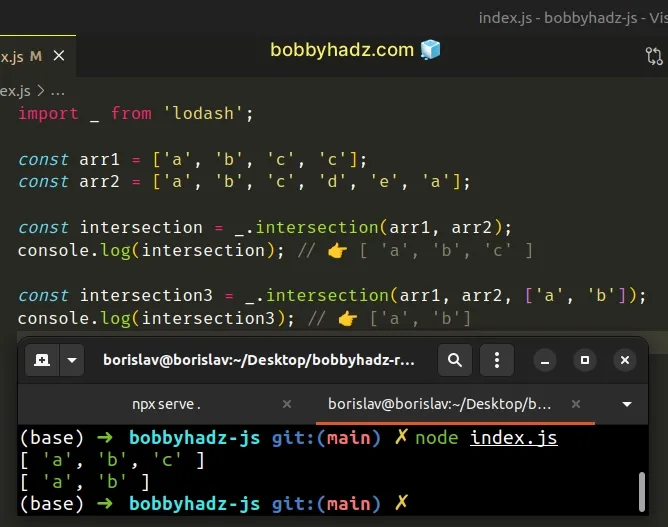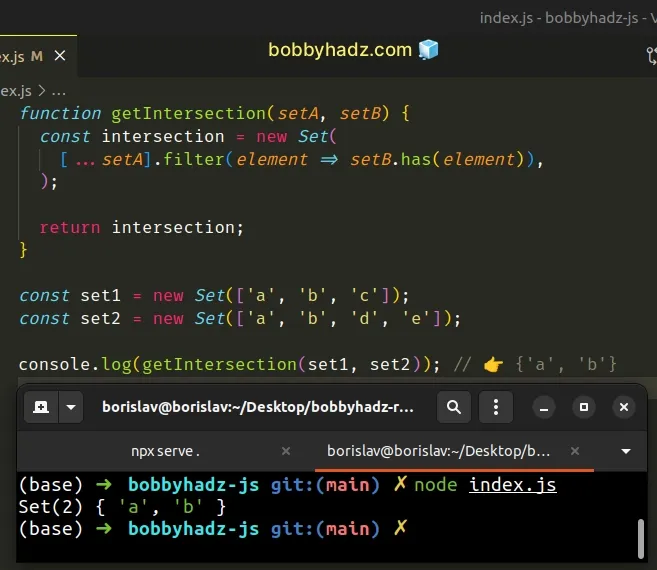# Get the Intersection of two Arrays or Sets in JavaScriptLast updated: Dec 27, 2022
5 min## #Get the Intersection of two Arrays in JavaScript

To get the intersection of two arrays:

1. Convert the arrays to `Set` objects to remove the duplicates.
2. Convert the first `Set` back to an array and call the `filter()` method on it.
3. On each iteration, check if the current element is contained in the second `Set`.
index.js
```Copied!```function getIntersection(a, b) {
const set1 = new Set(a);
const set2 = new Set(b);

const intersection = [...set1].filter(
element => set2.has(element)
);

return intersection;
}

const arr1 = ['a', 'b', 'c', 'c'];
const arr2 = ['a', 'b', 'c', 'd', 'e', 'a'];

// 👇️ ['a', 'b','c']
console.log(getIntersection(arr1, arr2));
``````We used the Set object to remove all the duplicates from the two arrays.

The `Set` object only stores unique values, so converting the array to a `Set` automatically removes all duplicates.
index.js
```Copied!```const set1 = new Set(['a', 'a', 'a']);

console.log(set1); // 👉️ Set(1) { 'a' }
``````

We did this because we don't want our intersection to contain duplicate values, e.g. `['a', 'b', 'c', 'c']`.

If you want your intersection to contain duplicate values (if there are any), use the `filter()` method directly.

index.js
```Copied!```const arr1 = ['a', 'b', 'c', 'c'];
const arr2 = ['a', 'b', 'c', 'd', 'e', 'a'];

const intersection = arr1.filter(element => arr2.includes(element));
console.log(intersection); // 👉️ [ 'a', 'b', 'c', 'c' ]
``````

You can also use two calls to the `Array.filter()` method to remove the duplicates from the intersection array.

index.js
```Copied!```const arr1 = ['a', 'b', 'c', 'c'];
const arr2 = ['a', 'b', 'c', 'd', 'e', 'a'];

const intersection2 = arr1
.filter(element => arr2.includes(element))
.filter((element, index, arr3) => arr3.indexOf(element) === index);

// 👇️ [ 'a', 'b', 'c' ]
console.log(intersection2);
``````

We then used the spread syntax (...) to convert the `Set` back to an array and called the `filter()` method on the array.

index.js
```Copied!```function getIntersection(a, b) {
const set1 = new Set(a);
const set2 = new Set(b);

const intersection = [...set1].filter(
element => set2.has(element)
);

return intersection;
}

const arr1 = ['a', 'b', 'c', 'c'];
const arr2 = ['a', 'b', 'c', 'd', 'e', 'a'];

// 👇️ ['a', 'b','c']
console.log(getIntersection(arr1, arr2));
``````

The function we passed to the Array.filter method gets called with each element of the array.

On each iteration, we check if the current element is contained in the second `Set`.

The `filter()` method returns an array containing the elements for which the test function returns a truthy value.

In our case, these are all the elements that are contained in both arrays.

You can also use the `Array.reduce()` method.

## #Get the Intersection of two or more Arrays using `reduce()`

This is a three-step process:

1. Use the `Array.reduce()` method to iterate over the arrays.
2. Use the `filter()` method to iterate over each array.
3. Check if each element is contained in the other array.
index.js
```Copied!```const arr1 = ['a', 'b', 'c', 'c'];
const arr2 = ['a', 'b', 'c', 'd', 'e', 'a'];
const arr3 = ['a', 'b'];

const arrays = [arr1, arr2, arr3];

const intersection = arrays.reduce((accumulator, array) => {
return accumulator.filter(element => array.includes(element));
});

console.log(intersection);
``````We stored the arrays in a list and used the `Array.reduce()` method to iterate over the two-dimensional list.

On each iteration, we use the `Array.filter()` method to iterate over the accumulated array and check if all of the elements in the array are contained in the next array.

The `Array.reduce()` method returns a new array that only contains the elements that are contained in all of the supplied arrays.

## #Get the Intersection of two or more Arrays using lodash

You can also use the `lodash` library.

1. Install the `lodash` library.
shell
```Copied!```npm install lodash

# 👇️ only if you use TypeScript
npm install @types/lodash --save-dev
``````
1. Use the `intersection()` method to get the intersection of two or more arrays.
index.js
```Copied!```import _ from 'lodash';

const arr1 = ['a', 'b', 'c', 'c'];
const arr2 = ['a', 'b', 'c', 'd', 'e', 'a'];

const intersection = _.intersection(arr1, arr2);
console.log(intersection); // 👉️ [ 'a', 'b', 'c' ]

const intersection3 = _.intersection(arr1, arr2, ['a', 'b']);
console.log(intersection3); // 👉️ ['a', 'b']
``````The `intersection()` method from `lodash` creates an array of unique values that are included in all of the provided arrays.

The method can be used to get the intersection of two or more arrays.

The method returns a new array containing the shared values of the supplied arrays.

## #Get the Intersection of two Sets in JavaScript

To get the intersection of two Sets:

1. Convert the first `Set` to an array.
2. Use the `filter()` method to iterate over the array.
3. Use the `has()` method to check if each value is contained in the second `Set`.
4. Convert the array back to a `Set`.
index.js
```Copied!```function getIntersection(setA, setB) {
const intersection = new Set(
[...setA].filter(element => setB.has(element))
);

return intersection;
}

const set1 = new Set(['a', 'b', 'c']);
const set2 = new Set(['a', 'b', 'd', 'e']);

console.log(getIntersection(set1, set2)); // 👉️ {'a', 'b'}
``````We used the spread syntax (...) to convert the first `Set` to an array and called the `Array.filter()` method on the array.

index.js
```Copied!```console.log([...set1]); // 👉️ ['a', 'b', 'c']
``````

The function we passed to the Array.filter method gets called with each element in the array.

The `filter()` method returns a new array that only contains the elements that meet the condition.

On each iteration, we call the Set.has method to check if the value is contained in the second `Set`.

index.js
```Copied!```function getIntersection(setA, setB) {
const intersection = new Set(
[...setA].filter(element => setB.has(element))
);

return intersection;
}
``````

The `has()` method returns `true` if the value is found in the `Set` and `false` otherwise.

The `filter()` method returns a new array that only contains the values that are found in both `Set` objects, in other words, the intersection.

The last step is to use the Set() constructor to convert the array back to a `Set`.

The `Set()` constructor takes an iterable object, such as an array, and adds all of its values into a newly created `Set`.

Finding the intersection of two `Set` objects is quite straightforward because `Set` objects contain no duplicate values.

## #Find the Intersection of two Set objects using `for...of`

This is a three-step process:

1. Use a `for...of` loop to iterate over the first `Set`.
2. Check if each element is contained in the second `Set`.
3. If the condition is met, add the element to a new `Set`.
index.js
```Copied!```function getIntersection(setA, setB) {
const intersection = new Set();

for (const element of setA) {
if (setB.has(element)) {
}
}

return intersection;
}

const set1 = new Set(['a', 'b', 'c']);
const set2 = new Set(['a', 'b', 'd', 'e']);

console.log(getIntersection(set1, set2)); // 👉️ {'a', 'b'}
``````

We declared a new variable and initialized it to an empty `Set`.

The for...of statement is used to loop over iterable objects like arrays, strings, `Map`, `Set` and `NodeList` objects and `generators`.

On each iteration, we check if the current element (of `setA`) is contained in `setB`.

If the condition is met, we add the element to the new `Set` object.

The Set.add method inserts a new element with the supplied value into a `Set` object, if the value is not already in the `Set`.